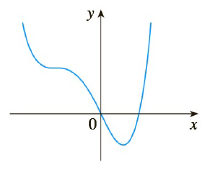Chapter 2.2, Problem 10E

Chapter
Section
Textbook Problem
3 views

# Trace or copy the graph of the given function f. (Assume that the axes have equal scales.) Then use the method of Example 1 to sketch the graph of f ′ below it.To determine

To sketch: The graph of f’ below the graph of the given function using the method of Example 1.

Explanation

Information used:

A tangent line is a line that touches the graph but does not intersect it at that point.

The slope of a horizontal tangent line is zero.

Formula used:

slope =  y2-y1x2-x1

Calculation:

The tangents at x = -2 and x = 1 are horizontal, so the derivative at those points is zero and the graph of derivative crosses the x axis at those points.

Here, f’(-2) = 0 and f’(1) = 0

In given graph, in the interval (-∞, -2) draw tangent at x = -2.5. The tangent passing through the points (-2.6, 2) and (-2.3, 1.4).

By using formula,

slope =  1.4-2-2.3+2.6= -2

Thus,

f-2.5= -2

Similarly, in the interval (-2, 1) draw tangent at x = -1

### Still sussing out bartleby?

Check out a sample textbook solution.

See a sample solution

#### The Solution to Your Study Problems

Bartleby provides explanations to thousands of textbook problems written by our experts, many with advanced degrees!

Get Started

#### Determine the infinite limit. limx3x(x3)5

Single Variable Calculus: Early Transcendentals, Volume I

#### Find the median for the following set of scores: 1, 9, 3, 6, 4, 3, 11, 10

Essentials of Statistics for The Behavioral Sciences (MindTap Course List)

#### Multiply: 1520320

Elementary Technical Mathematics

#### If n(A)=4, n(B)=5 and n(AB)=9, find n(AB).

Finite Mathematics for the Managerial, Life, and Social Sciences